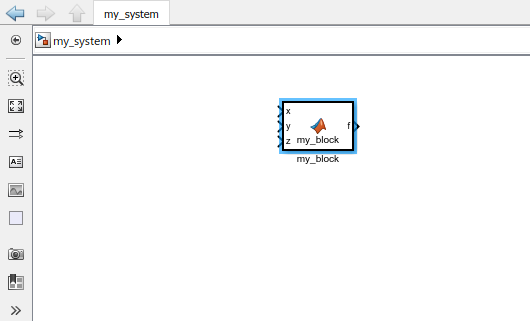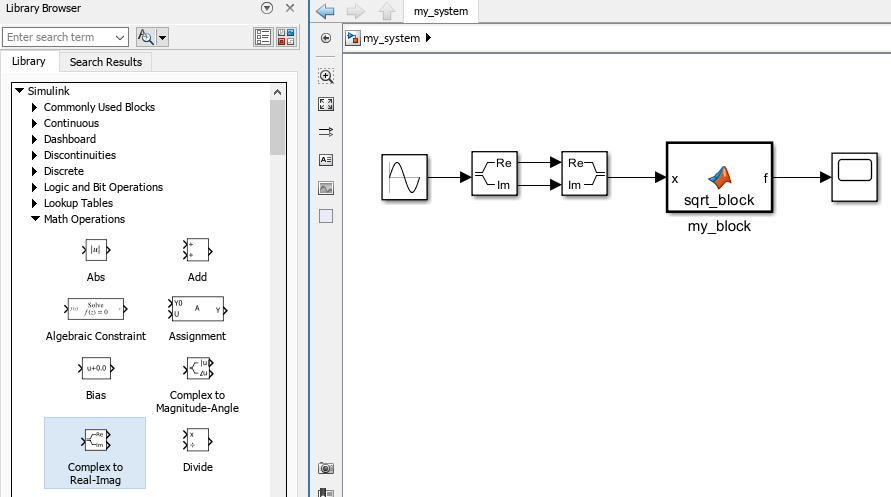# matlabFunctionBlock

Convert symbolic expression to MATLAB function block

## Syntax

``matlabFunctionBlock(block,f)``
``matlabFunctionBlock(block,f1,...,fN)``
``matlabFunctionBlock(___,Name,Value)``

## Description

example

````matlabFunctionBlock(block,f)` converts `f` to a MATLAB® function block that you can use in Simulink® models. Here, `f` can be a symbolic expression, function, or a vector of symbolic expressions or functions.`block` specifies the name of the block that you create or modify.```

example

````matlabFunctionBlock(block,f1,...,fN)` converts symbolic expressions or functions `f1,...,fN` to a MATLAB function block with `N` outputs. Each element of `f1,...,fN` can be a symbolic expression, function, or a vector of symbolic expressions or functions.```

example

````matlabFunctionBlock(___,Name,Value)` converts a symbolic expression, function, or a vector of symbolic expressions or functions to a MATLAB function block using additional options specified by one or more name-value arguments with any of the input argument combinations in the previous syntaxes.```

## Examples

collapse all

Create a new model and convert a symbolic expression to a MATLAB function block. Include comments in the block by specifying the `Comments` option.

Create a new model and open it.

```new_system('my_system') open_system('my_system')```

Create a symbolic expression.

```syms x y z f = x^2 + y^2 + z^2;```

Use `matlabFunctionBlock` to create the block `my_block` containing the symbolic expression. `matlabFunctionBlock` overwrites existing blocks.

`matlabFunctionBlock('my_system/my_block',f)`Double-click the generated block to open and edit the function defining the block.

```function f = my_block(x,y,z) %#codegen % This function was generated by the Symbolic Math Toolbox version 7.3. % 01-Jan-2017 00:00:00 f = x.^2+y.^2+z.^2;```

Include the comment `Version 1.1` in the block.

`matlabFunctionBlock('my_system/my_block',f,'Comments','Version: 1.1')`
```function f = my_block(x,y,z) ... %Version: 1.1 f = x.^2+y.^2+z.^2;```

Save and close `my_system`.

```save_system('my_system') close_system('my_system')```

Create a new model and convert a symbolic function to a MATLAB function block.

Create a new empty model and open it.

```new_system('my_system') open_system('my_system')```

Create a symbolic function.

```syms x y z f(x,y,z) = x^2 + y^2 + z^2;```

Convert `f` to a MATLAB function block. Double-click the block to see the function.

`matlabFunctionBlock('my_system/my_block',f)`
```function f = my_block(x,y,z) %#codegen f = x.^2+y.^2+z.^2;```

Convert several symbolic expressions to a MATLAB function block with multiple output ports.

Create a new empty model and open it.

```new_system('my_system') open_system('my_system')```

Create three symbolic expressions.

```syms x y z f = x^2; g = y^2; h = z^2;```

Convert them to a MATLAB function block. `matlabFunctionBlock` creates a block with three output ports. Double-click the block to see the function.

`matlabFunctionBlock('my_system/my_block',f,g,h)`
```function [f,g,h] = my_block(x,y,z) %#codegen f = x.^2; if nargout > 1 g = y.^2; end if nargout > 2 h = z.^2; end```

Specify the name of the function defining the generated MATLAB function block.

Create a new empty model and open it.

```new_system('my_system') open_system('my_system')```

Create a symbolic expression.

```syms x y z f = x^2 + y^2 + z^2;```

Generate a block and set the function name to `my_function`. Double-click the block to see the function.

```matlabFunctionBlock('my_system/my_block',f,... 'FunctionName','my_function')```
```function f = my_function(x,y,z) %#codegen f = x.^2+y.^2+z.^2;```

When you convert a symbolic expression to a MATLAB function block, `matlabFunctionBlock` optimizes the code by default. This approach can help simplify and speed up further computations that use the file. Nevertheless, generating the optimized code from some symbolic expressions and functions can be very time-consuming. Use `Optimize` to disable code optimization.

Create a new empty model and open it.

```new_system('my_system') open_system('my_system')```

Create a symbolic expression.

```syms x r = x^2*(x^2 + 1);```

Use `matlabFunctionBlock` to create the block `my_block` containing the symbolic expression. Double-click the block to see the function defining the block. By default, `matlabFunctionBlock` creates a file containing the optimized code.

`matlabFunctionBlock('my_system/my_block',r)`
```function r = my_block(x) %#codegen t2 = x.^2; r = t2.*(t2+1.0);```

Disable the code optimization by setting the value of `Optimize` to `false`.

```matlabFunctionBlock('my_system/my_block',r,... 'Optimize',false)```
```function r = my_block(x) %#codegen r = x.^2.*(x.^2+1.0);```

Specify the order of the input variables that form the input ports in a generated block.

Create a new empty model and open it.

```new_system('my_system') open_system('my_system')```

Create a symbolic expression.

```syms x y z f = x^2 + y^2 + z^2;```

Convert the expression to a MATLAB function block. By default, `matlabFunctionBlock` uses alphabetical order of input arguments when converting symbolic expressions.

`matlabFunctionBlock('my_system/my_block',f)`
```function f = my_block(x,y,z) %#codegen f = x.^2+y.^2+z.^2;```

Use the `Vars` argument to specify the order of the input ports.

```matlabFunctionBlock('my_system/my_block',f,... 'Vars',[y z x])```
```function f = my_block(y,z,x) %#codegen f = x.^2+y.^2+z.^2;```

Specify the input variables explicitly for automatically generated elements.

Create a new empty model and open it.

```new_system('my_system') open_system('my_system')```

Create a symbolic variable `u` and a 3-by-1 vector of symbolic variables `x`. The following command also automatically generates the symbolic variables `x1`, `x2`, and `x3` in the MATLAB workspace.

```syms u syms x [3 1] whos```
``` Name Size Bytes Class Attributes u 1x1 8 sym x 3x1 8 sym x1 1x1 8 sym x2 1x1 8 sym x3 1x1 8 sym ```

Create a symbolic expression that uses the symbolic variables `u` and `x`.

```A = [0 1 0; 0 0 1; -1 -1 -2]; b = [0; 0; 3]; xdot = A*x+b*u```
```xdot = x2 x3 3*u - x1 - x2 - 2*x3```

Convert the `xdot` expression to a MATLAB function block. Because the elements of `xdot` contain the variable `u` and the automatically generated variables `x1`, `x2`, and `x3`, specify the `Vars` argument in terms of these variables explicitly instead of using `u` and `x`.

`matlabFunctionBlock('my_system/my_block',xdot,'Vars',{u,x1,x2,x3})`
```function xdot = my_block(u,x1,x2,x3) %#codegen xdot = [x2;x3;u.*3.0-x1-x2-x3.*2.0];```

When generating a block, rename the output variables and the corresponding ports.

Create a new empty model and open it.

```new_system('my_system') open_system('my_system')```

Create a symbolic expression.

```syms x y z f = x^2 + y^2 + z^2;```

Convert the expression to a MATLAB function block and specify the names of the output variables and ports. Double-click the block to see the function defining the block.

```matlabFunctionBlock('my_system/my_block',f,f + 1,f + 2,... 'Outputs',{'name1','name2','name3'})```
```function [name1,name2,name3] = my_block(x,y,z) %#codegen t2 = x.^2; t3 = y.^2; t4 = z.^2; name1 = t2+t3+t4; if nargout > 1 name2 = t2+t3+t4+1.0; end if nargout > 2 name3 = t2+t3+t4+2.0; end```

Call `matlabFunctionBlock` using several name-value pair arguments simultaneously.

Create a new empty model and open it.

```new_system('my_system') open_system('my_system')```

Create a symbolic expression.

```syms x y z f = x^2 + y^2 + z^2;```

Call `matlabFunctionBlock` using the name-value pair arguments to specify the function name, the order of the input ports, and the names of the output ports. Double-click the block to see the function defining the block.

```matlabFunctionBlock('my_system/my_block',f,f + 1,f + 2,... 'FunctionName','my_function','Vars',[y z x],... 'Outputs',{'name1','name2','name3'})```
```function [name1,name2,name3] = my_function(y,z,x) %#codegen t2 = x.^2; t3 = y.^2; t4 = z.^2; name1 = t2+t3+t4; if nargout > 1 name2 = t2+t3+t4+1.0; end if nargout > 2 name3 = t2+t3+t4+2.0; end```

Specify a complex input in the generated MATLAB function block.

Create a new empty model and open it.

```new_system('my_system') open_system('my_system')```

Create a symbolic function that finds the square root of an input.

```syms x f = sqrt(x);```

Convert `f` to a MATLAB function block.

`matlabFunctionBlock('my_system/my_block',f,'FunctionName','sqrt_block')`

Because the square root function generates a complex result when the input `x` is negative, you need to manually specify a complex input in the generated block. Otherwise, you can encounter an error when running the function block with a negative input.

Double-click the block to see the function defining the block. Add the line `x = complex(x);` to specify a complex input. Starting in R2023a, `matlabFunctionBlock` provides this line and you can uncomment it. The generated function block now accepts a complex input and returns a complex output.

```function f = sqrt_block(x) %#codegen x = complex(x); % required to enforce complex operations on x f = sqrt(x); end```

As an alternative, you can also insert the Complex to Real-Imag (Simulink) and Real-Imag to Complex (Simulink) blocks from the Simulink > Math Operations library. Add these blocks to convert the input signal that goes into `x` to a complex signal.## Input Arguments

collapse all

Block to create or modify, specified as a character vector.

Symbolic input to be converted to a MATLAB function block, specified as a symbolic expression, function, vector, or matrix.

Symbolic input to be converted to a MATLAB function block with `N` outputs, specified as several symbolic expressions, functions, vectors, or matrices, separated by commas.

### Name-Value Arguments

Specify optional pairs of arguments as `Name1=Value1,...,NameN=ValueN`, where `Name` is the argument name and `Value` is the corresponding value. Name-value arguments must appear after other arguments, but the order of the pairs does not matter.

Before R2021a, use commas to separate each name and value, and enclose `Name` in quotes.

Example: `matlabFunctionBlock('my_system/my_block',f,'FunctionName','myfun')`

Comments to include in the file header, specified as a character vector, cell array of character vectors, or string vector.

Name of the function, specified as a character vector. By default, `matlabFunction(block,…)` uses `block` as the function name.

Flag for code optimization, specified as `true` or `false`.

When writing to a file, `matlabFunctionBlock` optimizes the code using intermediate variables named `t0`, `t1`, ....

Order of input variables and corresponding input ports of the generated block, specified as a character vector, a vector of symbolic variables, or a one-dimensional cell array of character vectors, symbolic variables, or vectors of symbolic variables.

The number of specified input ports must equal or exceed the number of free variables in `f`. Do not use the same names for the input ports specified by `Vars` and the output ports specified by `Outputs`.

By default, when you convert symbolic expressions, the order is alphabetical. When you convert symbolic functions, their input arguments appear in front of other variables, and all other variables are sorted alphabetically.

Names of output ports, specified as a one-dimensional cell array of character vectors. If you do not specify the output port names, `matlabFunctionBlock` uses names that consist of the word `out` followed by output port numbers, for example, `out3`.

Do not use the same names for the input ports specified by `Vars` and the output ports specified by `Outputs`. See Specify Output Ports.

## Limitations

• Some symbolic functions that have no corresponding MATLAB functions operating on the `double` data type, such as `simplify` and `solve`, are kept as symbolic functions in the generated MATLAB function block. The converted function block that consists of these functions cannot be used in Simulink models. You need to create your own functions with the `double` data type to replace these symbolic functions. If you are interested in a symbolic function that cannot be used in Simulink models, please contact MathWorks Technical Support.

## Version History

Introduced in R2009a